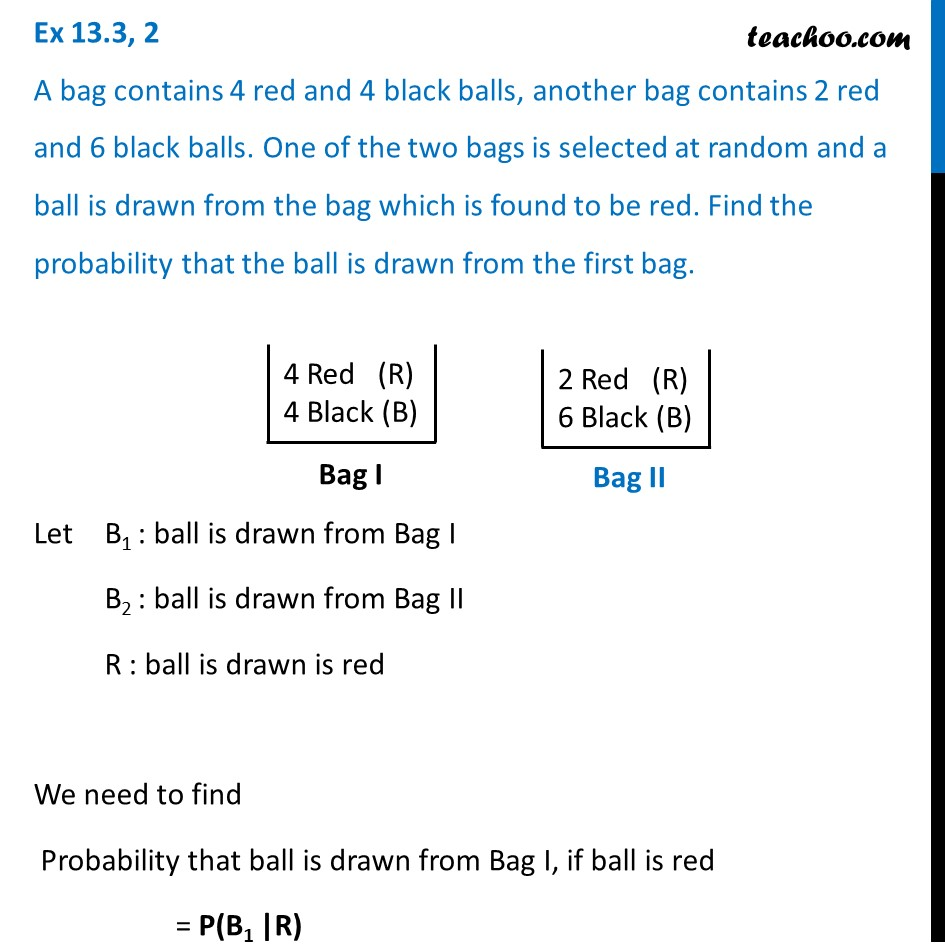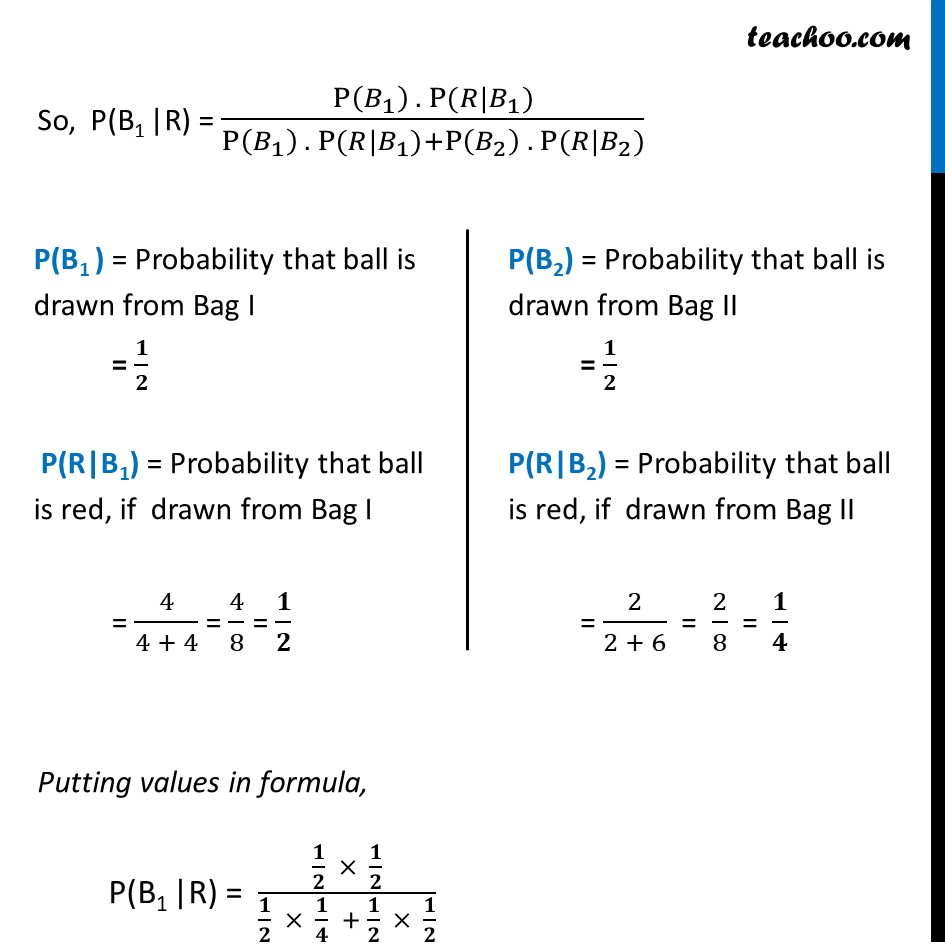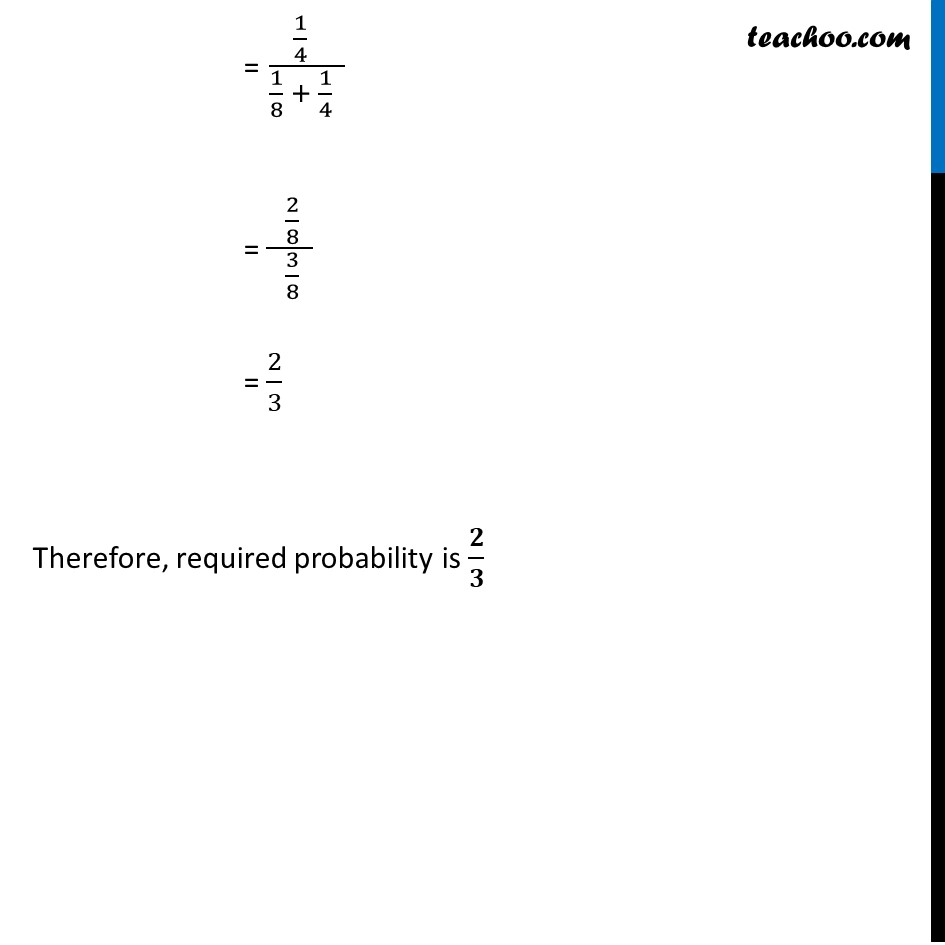Chapter 13 Class 12 Probability

Class 12
Important Questions for exams Class 12Learn in your speed, with individual attention - Teachoo Maths 1-on-1 Class

### Transcript

Ex 13.3, 2 A bag contains 4 red and 4 black balls, another bag contains 2 red and 6 black balls. One of the two bags is selected at random and a ball is drawn from the bag which is found to be red. Find the probability that the ball is drawn from the first bag.4 Red (R) 4 Black (B) 2 Red (R) 6 Black (B) Let B1 : ball is drawn from Bag I B2 : ball is drawn from Bag II R : ball is drawn is red We need to find Probability that ball is drawn from Bag I, if ball is red = P(B1 |R) So, P(B1 |R) = (P(𝐵_1 ) . P(𝑅|𝐵_1))/(P(𝐵_1 ) . P(𝑅|𝐵_1)+P(𝐵_2 ) . P(𝑅|𝐵_2)) "P(B1 )" = Probability that ball is drawn from Bag I = 𝟏/𝟐 "P(R|B1)" = Probability that ball is red, if drawn from Bag I = 4/(4 + 4) = 4/8 = 𝟏/𝟐 "P(B1 )" = Probability that ball is drawn from Bag I = 𝟏/𝟐 "P(R|B1)" = Probability that ball is red, if drawn from Bag I = 4/(4 + 4) = 4/8 = 𝟏/𝟐 Putting values in formula, P(B1 |R) = (𝟏/𝟐 × 𝟏/𝟐)/(𝟏/𝟐 × 𝟏/𝟒 + 𝟏/𝟐 × 𝟏/𝟐) = (1/4 )/(1/8 + 1/4 ) = ( 2/8 )/( 3/8 ) = 2/3 Therefore, required probability is 𝟐/𝟑